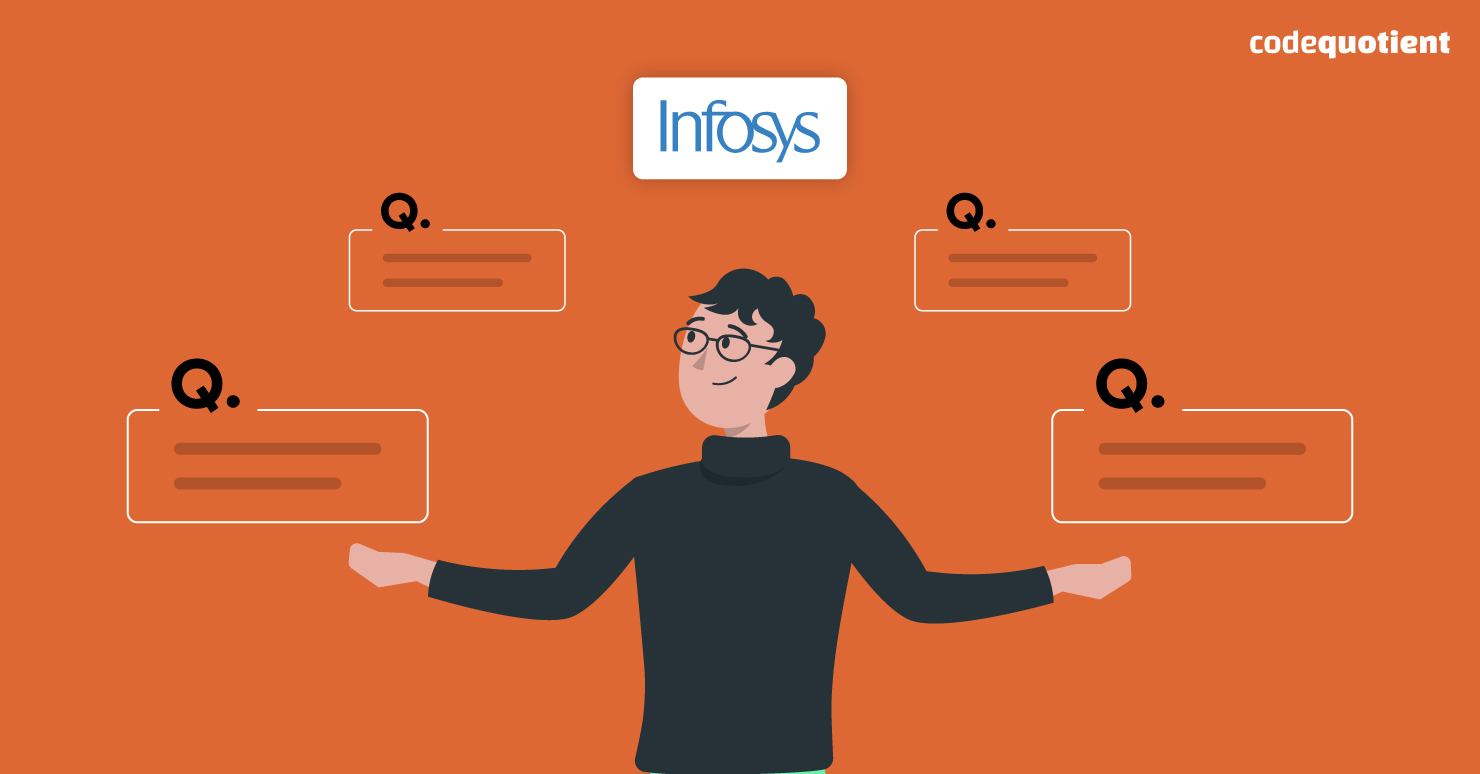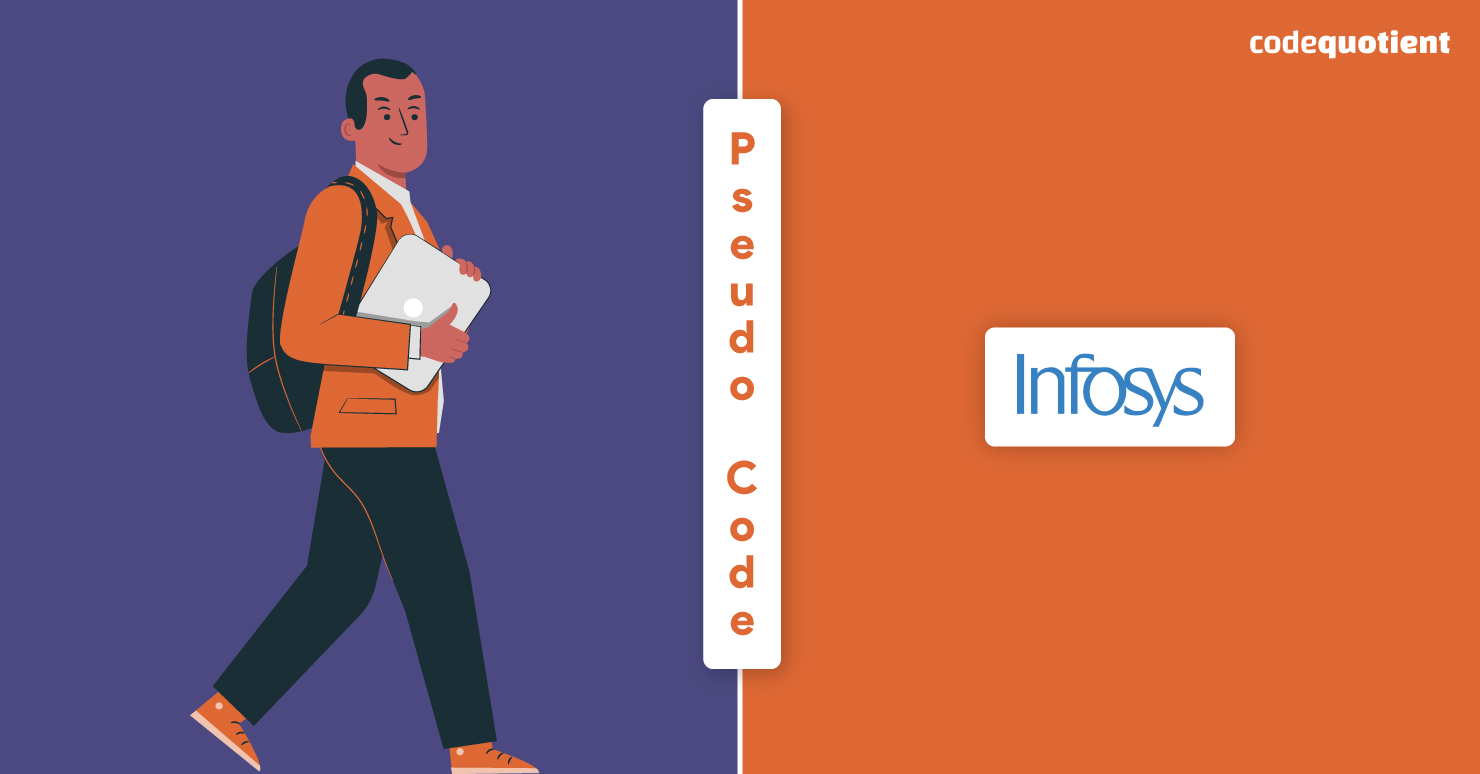If you are preparing for Infosys and must go through pseudocode questions quickly, you’ve arrived at the perfect place. A good practice of the most often asked questions is what you require to ace the interview with Infosys coding questions.

And after a lot of brainstorming, we are here with 6 of the best pseudocode questions for Infosys that will help you shine and get hired.

## What Do You Mean by Pseudocode?

Now before moving to the questions, let us clear our basics. A PseudoCode is a simplified notation language that helps developers build algorithms. Simply put, it is a code that even humans can read, not just machines. This implies that even the Pseudocode questions for Infosys will be quite easy, and one could learn them in a short span of time. With this in mind, let us move forward to the questions with a boost of confidence and adrenaline.

### Is Pseudocode Important in Infosys coding questions?Infosys has a multi-level test series that finalises the selection of any candidate. The pseudocode test is the fourth level of the test series. A duration of ten minutes is given to solve five questions of pseudocode, making it pretty hard as the candidate only gets a maximum of two minutes per question. Keeping in mind that only the candidate who passes all the levels is ultimately eligible for the puzzle-solving round and, finally, the job interview, the pseudocode test is very important.

## 6 Infosys Coding Questions on Pseudocode

It is always good to prepare with similar questions to clear the level easily and within the time frame. Solve as many questions as possible, and do not miss out on the 6 best pseudocode questions below for Infosys 2023.

### 1. Give Output to the following Code

#include

int main()

{

typedef int num;

num bunk = 0.00;

printf(“%d”, bunk);

return 0;

}

Options:

1. 0
2. 0.0
3. Error
4. None of the above

Answer: The correct answer will be option a) 0. In C language, %d denotes an integer, and the integer part of 0.0 is 0. And that is why from printf(“%d, bunk) only 0 will print.

### 2. What is the Possible Output of the Given Code?

#include

int main()

{

int go = 5.0, num = 1*10;

do

{

num /= go;

} while(go–);

printf (“%d\n”, num);

return 0;

}

Options:

1. No Output
2. Compilation Error
3. Floating Pointer Exception
4. 5 10 15

Answer: The correct option is c) Floating Pointer Exception. This is because, while writing a code in C language, if an integer is divided with a float without any type casting, then it gives the error – “Floating Point Exception”.

### 3. What Will Be the Outcome of the Following Code?

#include

int main()

{

int num = 987;

int rem;

while(num!=0)

{

rem = num % 4;

num = num / 10;

}

printf(“%d”,rem);

}

Options:

1. 0
2. 1
3. 10
4. 5

Answer: The correct option is b) 1. The explanation is quite simple. The first iteration, rem = 3(987 % 4) has num = 98. The second Iteration, rem = 2(98 % 4) has num = 9. Therefore the third Iteration, rem = 1(9 % 4) will give num = 1.

### 4. Give Output of the Following Program

public class Main

{

public static void main(String[] args)

{

String names[] = new String;

for(int x=0; x

Options:

1. Exception
2. Null
3. None
4. Compilation Error
Also Read:  Best Hands-on Summer Training for CSE Students

Answer: The correct option is b) Null. Since the value of args.length is 0, the loop will not run the time. Therefore, Null will get printed.

### 5. Predict the Output for the Given Code

#include

Int main()

{

float x = 0.0;

long int y = 10;

printf(“%d”, sizeof(y) == sizeof(x+y));

return 0;

}

Options:

1. 3
2. 1
3. 5
4. 2

Answer: The correct option is b) 1. While coding in the C language, the size of variable x of type float is 4. The size of variable y that is long int is 8. On combining both i.e., (x + y) the size results in a float, which is again 4. Since the question asks: the size of(y) == size of(x+y) which we know is 4 == 4. Then the operator “==” returns either 0 or 1, so the correct answer is 1.

### 6. Predict the Output

#include

int main()

{

int x;

for (x = 10; x >= 0; x–) {

int z = x & (x >> 1);

if (z)

printf(“%d “, x);

}

}

Options:

1. 678
2. 763
3. 6 7 8
4. 7 6 3

Answer: The correct option is d) 7 6 3. The value of z will be 1 for x = 7, 6, and 3 where z is non-zero. So the values 7, 6, and 3 will print on the screen.

## Conclusion

If you try to wade in too quickly and start coding on the whiteboard, you’ll find yourself struggling to implement a solution for a question you know little about. This may work for some knowledge questions where it’s not vital to have an understanding of why something works; however, this isn’t the case for the Infosys coding questions.

Also Read:  TCS Digital Questions to Improve Your Reasoning Ability for the 2023 Exam

The exclusive free SuperCoders Program can help anyone looking for hands-on practice with full-stack development paid Internship. Their unique focus on internship helps the learner grasp and retain things fundamentally and for a long time. Also, visit their website to learn more about CodeQuotient, which is associated with colleges.

## Get UGC Approved BCA DegreeAnd Earn While You Do That

Join CodeQuotient's Software Engineering Program

Study at NAAC A+ Accredited Partner University